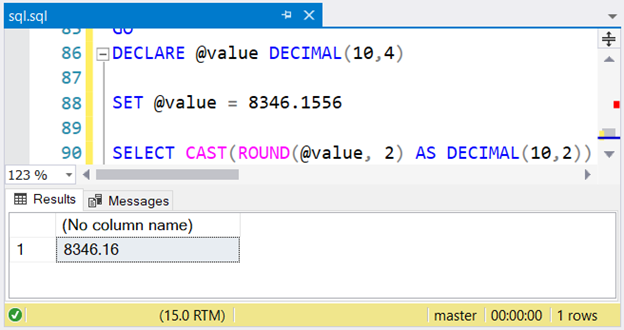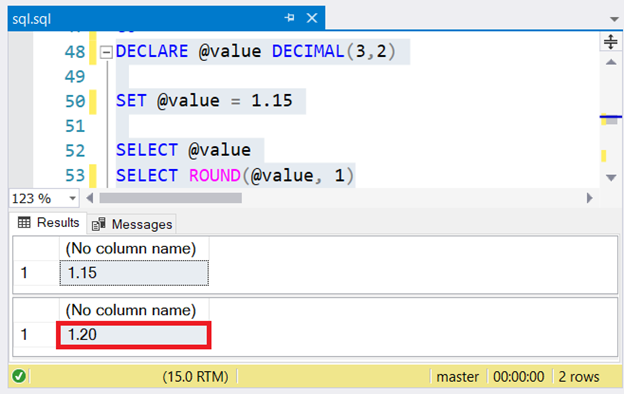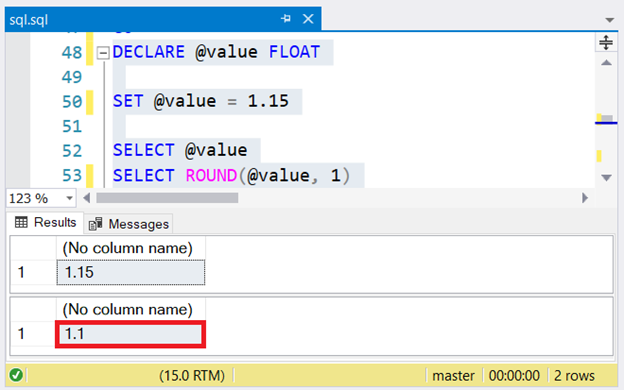15:15

# How to Round (UP/DOWN) in SQL Server – 5 Useful TipsWe’ve been taught to round numbers since we were kids. When you round off 1.15 to the nearest tenths, will it be 1.2 or 1.1? Using the SQL ROUND function to answer may confuse you. Later, you will see what I mean.

[sendpulse-form id=”12010″]

Here’s another question. What’s the sum of 1 + 1? That’s quite a stupid question. It’s for kids learning math for the first time, not for us grown-ups. But please have a look at the code below:

``````-- Variables for input values
DECLARE @value1 DECIMAL(3,2) = 1.05
DECLARE @value2 DECIMAL(3,2) = 1.45
DECLARE @sum1 DECIMAL(3,2) = @value1 + @value2

-- Variables for rounded values
DECLARE @roundedValue1 TINYINT = ROUND(@value1,0)
DECLARE @roundedvalue2 TINYINT = ROUND(@value2,0)
DECLARE @sum2 TINYINT = ROUND(@sum1,0)

-- Surprise!
SELECT 'sum of ' + CAST(@value1 AS VARCHAR(4)) + ' + ' + CAST(@value2 AS VARCHAR(4)) AS Q1,  @sum1 AS Sum1
SELECT 'sum of ' + CAST(@roundedValue1 AS VARCHAR(4)) + ' + ' + CAST(@roundedValue2 AS VARCHAR(4)) AS Q2,  @sum2 AS Sum2
``````

Then check the results below:

What’s happened here? We get two values with a decimal part. Then, we round them using SQL ROUND to the nearest whole number. Their sums are rounded too. But how can 1 + 1 be 3?!

A sharp-eyed SQL pro will spot the problem in the code at once. But consider this:

• Rounding 2.5 to the nearest whole number is 3, not 2.
• The sum of 1 + 1 is 2.

It’s hard, isn’t it?

Here’s the point. If you are not careful, SQL ROUND can make you nuts. This can also be a source of arguments between developers and accountants. One of these is when dealing with the materiality principle. The arguments can get ugly.

What can you do?

## Round Function in SQL Server

No, I’m not telling you to pass this problem to the next developer. This post will give you quintessential tips to make both you and your user happy with the result. Some of these tips can also apply to rounding a number on the app’s front-end side or in reports.

Let’s check our 5 tips in detail.

## Round off Function for Numbers in SQL

• Do you round off the input values BEFORE doing calculations?
• Or, do you calculate the input values, and then round off the result?

More questions can arise depending on the calculations you’re going to make.

The point is, whether you get the rounding off standard or model from the users. Then you can use this model to write queries. You don’t assume or guess which would lead to disagreement later. And if it helps to include this standard as a note somewhere in the report, do it.

Another part of the standard is how many decimal places to use. What if the table column has a data type of DECIMAL(10,4)? How will you round it to 2 decimal places only?

Try the code below:

``````DECLARE @value DECIMAL(10,4)

SET @value = 8346.1556

-- This will result in 8346.16 instead of 8346.1600
SELECT CAST(ROUND(@value, 2) AS DECIMAL(10,2))
``````

The CAST after the ROUND will display two decimal places only. The two zeroes will be truncated:Figure 2. Using CAST() after rounding to 2 decimal places of a number with 4 decimal places.

## UnderstandingHow SQL Round to Decimal Places

Here we answer our first question. When you round off 1.15 to the nearest tenths, will it be 1.2 or 1.1?

First, you check it with the DECIMAL data type:

``````DECLARE @value DECIMAL(3,2)

SET @value = 1.15

SELECT @value
SELECT ROUND(@value, 1)  -- This will result in 1.2 or 1.20
``````

The result of the code above is 1.20 or 1.2:Figure 3. Rounding off 1.15 to the nearest tenths results to 1.20 using a DECIMAL data type.

But what if the data type is a FLOAT? Let’s try to change it.

``````DECLARE @value FLOAT

SET @value = 1.15

SELECT @value
SELECT ROUND(@value, 1)  -- This will result to 1.1
``````

What is the result? It’s 1.1. See it for yourself:Figure 4. Rounding off 1.15 to the nearest tenths results to 1.1 using the FLOAT data type.

It is not that I want to give you more doubts. However, you need to know that these data types, though both used for numbers, are not created equal.

• FLOAT and REAL are approximate numerics, not recommended for rounding – not even for equality checks in a WHERE clause.
• DECIMAL and NUMERIC have fixed precision and scale, and we refer to them as exact numerics. Therefore, when we round off 1.15 to the nearest tenths, the correct answer is 1.2.
• Integers are also exact numerics. They are safe for rounding off whole digits.

The dilemma starts from the table design. You may want to do something about FLOAT or REAL columns that will be rounded off somewhere.

## 3. Use SQL ROUND on the Same Data Source for Consistency

Assume you need to create several revenue reports to show different details: the summary, the detailed breakdown by type, and the detailed breakdown by source. All these three reports will treat cents or decimal parts as immaterial. So, rounding values to whole numbers is inevitable.

For consistent results in all three reports, apply the following:

• SELECT from the same base tables to ensure that totals from the details are consistent with the summary.
• Use the same model for rounding off (point #1 above)

Do you want to end up happy with your users on these revenue reports? Be consistent on where and how you get your numbers.

## 4. SQLFLOORorCEILINGVSROUND

There may be cases where you must round up or round down to the next whole number. In this case, CEILING() or FLOOR() is the appropriate choice instead of ROUND()

CEILING() rounds up a value to the next integer:

``````SELECT CEILING(1);   -- returns 1
SELECT CEILING(1.6);  -- returns 2
SELECT CEILING(1.4);  -- returns 2
``````

Meanwhile, FLOOR()rounds down:

``````SELECT FLOOR(1);   -- returns 1
SELECT FLOOR(2.1); -- returns 2
SELECT FLOOR(2.9); -- returns 2
``````

## 5. Test the Results with your Users

After you complete all the queries and report design, the final part is to check your work with users. We can make mistakes no matter how good we are or how hard we work. Sometimes, we need a few more iterations to get it right.

Your users will have test scenarios that will ensure the accuracy of the reports. Or she may have an Excel file done manually to compare your work to theirs. In any case, work with them for a better system. You’ll be glad you did.

## Conclusion

Working with SQL ROUND can be hard at times. However, the tips presented here prove that you can win against these hurdles. What have you learned?

• Ask for the rounding off standard or model from your users.
• Know the data type you are using.
• Use ROUND on the same data source for consistency.
• Sometimes, FLOOR or CEILING might be appropriate than ROUND.